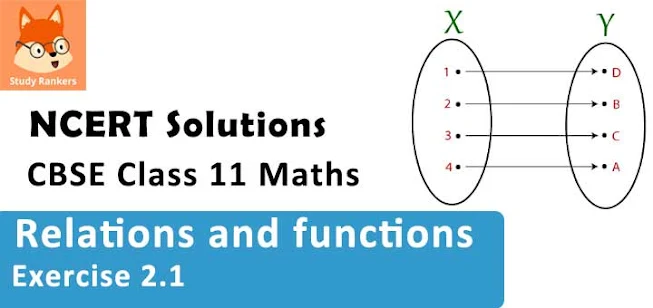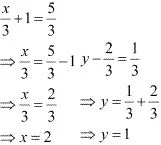# Class 11 Maths NCERT Solutions for Chapter 2 Relations and Functions Exercise 2.1### Relations and Functions Exercise 2.1 Solutions

1. If (x/3 + 1, y - 2/3) = (5/3 1/3) , find the values of x and y.

Solution

It is given that (x/3 + 1, y - 2/3) = (5/3, 1/3).
Since the ordered pairs are equal, the corresponding elements will also be equal.
Therefore, x/3 + 1= 5/3 and y - 2/3 = 1/3.2. If the set A has 3 elements and the set B = {3, 4, 5}, then find the number of elements in (A × B)?

Solution

It is given that set A has 3 elements and the elements of set B are 3, 4, and 5.
⇒ Number of elements in set B = 3
Number of elements in (A × B)
= (Number of elements in A) × (Number of elements in B)
= 3 × 3 = 9
Thus, the number of elements in (A × B) is 9.

3. If G = {7, 8} and H = {5, 4, 2}, find G × H and H × G.

Solution

G = {7, 8} and H = {5, 4, 2}
We know that the Cartesian product P × Q of two non-empty sets P and Q is defined as
P × Q = {(pq): p∈ P, q ∈ Q}
∴G × H = {(7, 5), (7, 4), (7, 2), (8, 5), (8, 4), (8, 2)}
H × G = {(5, 7), (5, 8), (4, 7), (4, 8), (2, 7), (2, 8)}

4. State whether each of the following statement are true or false. If the statement is false, rewrite the given statement correctly.
(i) If P = {mn} and Q = {nm}, then P × Q = {(mn), (nm)}.
(ii) If A and B are non-empty sets, then A × B is a non-empty set of ordered pairs (xy) such that x ∈ A and y ∈ B.
(iii) If A = {1, 2}, B = {3, 4}, then A × (B ∩ Φ) = Φ.

Solution

(i) False
If P = {mn} and Q = {nm}, then
P × Q = {(mm), (mn), (n, m), (nn)}

(ii) True

(iii) True

5. If A = {–1, 1}, find A × A × A.

Solution

It is known that for any non-empty set A, A × A × A is defined as
A × A × A = {(abc): ab∈ A}
It is given that A = {–1, 1}
∴ A × A × A = {(–1, –1, –1), (–1, –1, 1), (–1, 1, –1), (–1, 1, 1),
(1, –1, –1), (1, –1, 1), (1, 1, –1), (1, 1, 1)}

6. If A × B = {(ax), (ay), (bx), (by)}. Find A and B.

Solution

It is given that A × B = {(ax), (a, y), (bx), (by)}
We know that the Cartesian product of two non-empty sets P and Q is defined as P × Q {(pq): p ∈ P, q ∈ Q}
∴ A is the set of all first elements and B is the set of all second elements.
Thus, A = {ab} and B = {xy}

7. Let A = {1, 2}, B = {1, 2, 3, 4}, C = {5, 6} and D = {5, 6, 7, 8}. Verify that
(i) A × (B ∩ C) = (A × B) ∩ (A × C)
(ii) A × C is a subset of B × D

Solution

(i) To verify: A × (B ∩ C) = (A × B) ∩ (A × C)
We have B ∩ C = {1, 2, 3, 4} ∩ {5, 6} = Φ
∴L.H.S. = A × (B ∩ C) = A × Φ = Φ
A × B = {(1, 1), (1, 2), (1, 3), (1, 4), (2, 1), (2, 2), (2, 3), (2, 4)}
A × C = {(1, 5), (1, 6), (2, 5), (2, 6)}
∴ R.H.S. = (A × B) ∩ (A × C) = Φ
∴L.H.S. = R.H.S
Hence, A × (B ∩ C) = (A × B) ∩ (A × C)

(ii) To verify: A × C is a subset of B × D
A × C = {(1, 5), (1, 6), (2, 5), (2, 6)}
B × D = {(1, 5), (1, 6), (1, 7), (1, 8), (2, 5), (2, 6), (2, 7), (2, 8), (3, 5), (3, 6), (3, 7), (3, 8), (4, 5), (4, 6), (4, 7), (4, 8)}
We can observe that all the elements of set A × C are the elements of set B × D.
Therefore, A × C is a subset of B × D.

8. Let A = {1, 2} and B = {3, 4}. Write A × B. How many subsets will A × B have? List them.

Solution

A = {1, 2} and B = {3, 4}
∴A × B = {(1, 3), (1, 4), (2, 3), (2, 4)}
⇒ n(A × B) = 4
We know that if C is a set with n(C) = m, then n[P(C)] = 2m.
Therefore, the set A × B has 24 = 16 subsets. These are
Φ, {(1, 3)}, {(1, 4)}, {(2, 3)}, {(2, 4)}, {(1, 3), (1, 4)}, {(1, 3), (2, 3)},
{(1, 3), (2, 4)}, {(1, 4), (2, 3)}, {(1, 4), (2, 4)}, {(2, 3), (2, 4)},
{(1, 3), (1, 4), (2, 3)}, {(1, 3), (1, 4), (2, 4)}, {(1, 3), (2, 3), (2, 4)},
{(1, 4), (2, 3), (2, 4)}, {(1, 3), (1, 4), (2, 3), (2, 4)}

9. Let A and B be two sets such that n(A) = 3 and n (B) = 2. If (x, 1), (y, 2), (z, 1) are in A × B, find A and B, where xy and z are distinct elements.

Solution

It is given that n(A) = 3 and n(B) = 2; and (x, 1), (y, 2), (z, 1) are in A × B.
We know that A = Set of first elements of the ordered pair elements of A × B
B = Set of second elements of the ordered pair elements of A × B.
∴ xy, and z are the elements of A; and 1 and 2 are the elements of B.
Since n(A) = 3 and n(B) = 2, it is clear that A = {xyz} and B = {1, 2}.

10. The Cartesian product A × A has 9 elements among which are found (–1, 0) and (0, 1). Find the set A and the remaining elements of A × A.

Solution

We know that if n(A) = and n(B) = q, then n(A × B) = pq.
∴ n(A × A) = n(A) × n(A)
It is given that n(A × A) = 9
∴ n(A) × n(A) = 9
⇒ n(A) = 3
The ordered pairs (–1, 0) and (0, 1) are two of the nine elements of A × A.
We know that A × A = {(a, a): a ∈ A}. Therefore, –1, 0, and 1 are elements of A.
Since n(A) = 3, it is clear that A = {–1, 0, 1}.
The remaining elements of set A × A are (–1, –1), (–1, 1), (0, –1), (0, 0),
(1, –1), (1, 0), and (1, 1)